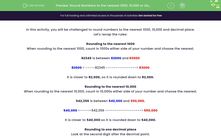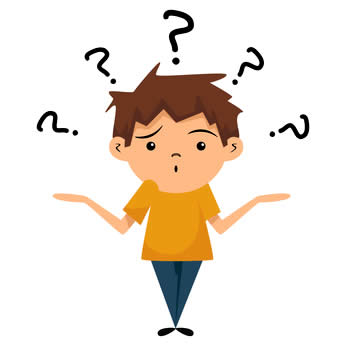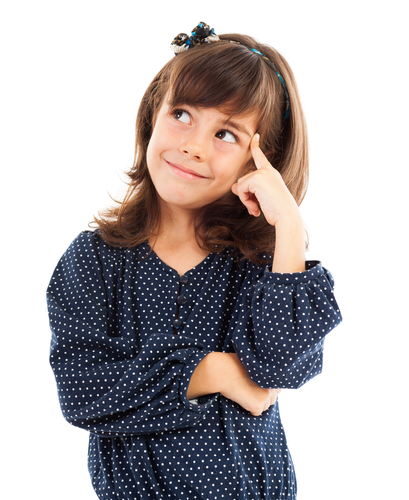# Round Numbers Including to One or Two Decimal Places

In this worksheet, students will be asked to round numbers to the nearest 1,000, 10,000 or to one or two decimal places.Key stage:  KS 2

Curriculum topic:   Number: Number and Place Value

Curriculum subtopic:   Round Numbers for Accuracy

Popular topics:   Decimals worksheets, Rounding worksheets, Estimation worksheets

Difficulty level:#### Worksheet Overview

In this activity, we will have the challenge of rounding numbers to the nearest 1,000, 10,000 and to one or two decimal places. So, a right mixture of challenges!Let's recap the rules:

Rounding to the nearest 1,000

When rounding to the nearest 1,000, count in 1,000s either side of your number and choose the nearest.

82,345 is between 82,000 and 83,000

82,000 < -----82,345 ----------------> 83,000

It is closer to 82,000, so it is rounded down to 82,000.

Rounding to the nearest 10,000

When rounding to the nearest 10,000, count in 10,000s either side of your number and choose the nearest.

542,356 is between 540,000 and 550,000.

540,000 <------542,356 -------------------> 550,000

It is closer to 540,000 so it is rounded down to 540,000.

Rounding to one decimal place

Look at the second digit after the decimal point.

So, for 8.42, we look at the 2, it is less than 5 so we leave the 4 to the left of it the same.

8.42 rounded to one decimal place is 8.4.

Rounding to two decimal places

Look at the third digit after the decimal place.

3.928...the third digit is 8 because it is more than 5, you round the 2 to the left of it, up to 3.

3.928 rounded to two decimal places is 3.93.

How do you feel about all that?Let's get started then. We can look back at this introduction at any point by clicking on the red help button that will appear on the screen when we start the questions.

### What is EdPlace?

We're your National Curriculum aligned online education content provider helping each child succeed in English, maths and science from year 1 to GCSE. With an EdPlace account you’ll be able to track and measure progress, helping each child achieve their best. We build confidence and attainment by personalising each child’s learning at a level that suits them.

Get started